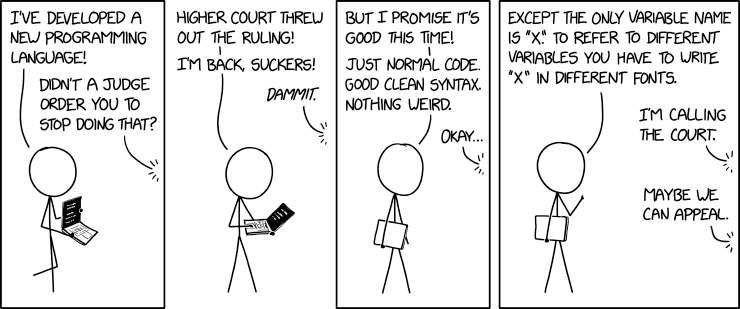# Basics of the Julia language

Author

Marie-Hélène Burle

Comments do not get evaluated by Julia and are for humans only.

``# Comments in Julia are identified by hastags``
``````#=
if you enclose them with this syntax
=#``````
``x = 2          # Comments can be added at the end of lines``
``2``

## Basic operations

``````# By default, Julia returns the output
2 + 3``````
``5``
``````# Trailing semi-colons suppress the output
3 + 7;``````
``````# Alternative syntax that can be used with operators
+(2, 5)``````
``7``
``````# Updating operators
a = 3
a += 8    # this is the same as a = a + 8``````
``11``
``````# Operator precedence follows standard rules
3 + 2 ^ 3 * 10``````
``83``

### More exotic operators

``````# Usual division
6 / 2``````
``3.0``
``````# Inverse division
2 \ 6``````
``3.0``
``````# Integer division (division truncated to an integer)
7 ÷ 2``````
``3``
``````# Remainder
7 % 2        # equivalent to rem(7, 2)``````
``1``
``````# Fraction
4//8``````
``1//2``
``````# Julia supports fraction operations
1//2 + 3//4``````
``5//4``

## Variablesfrom xkcd.com

A variable is a name bound to a value:

``a = 3;``

It can be called:

``a``
``3``

Or used in expressions:

``a + 2``
``5``

### Assignment

You can re-assign new values to variables:

``````a = 3;
a = -8.2;
a``````
``-8.2``

Even values of a different type:

``a = "a is now a string"``
``"a is now a string"``

You can define multiple variables at once:

``````a, b, c = 1, 2, 3
b``````
``2``

### Variable names

These names are extremely flexible and can use Unicode character:

``````\omega       # press TAB
\sum         # press TAB
\sqrt        # press TAB
\in          # press TAB
\:phone:     # press TAB``````
``````δ = 8.5;
🐌 = 3;
δ + 🐌``````
``11.5``

Admittedly, using emojis doesn’t seem very useful, but using Greek letters to write equations really makes Julia a great mathematical language:

``````σ = 3
δ = π
ϕ = 8

(5σ + 3δ) / ϕ``````
``3.0530972450961724``

Note how the multiplication operator can be omitted when this does not lead to confusion.
Also note how the mathematical constant π is available in Julia without having to load any module.

If you want to know how to type a symbol, ask Julia: type `?` and paste it in the REPL.

The only hard rules for variable names are:

• They must begin with a letter or an underscore,
• They cannot take the names of built-in keywords such as `if`, `do`, `try`, `else`,
• They cannot take the names of built-in constants (e.g. `π`) and keywords in use in a session.

We thus get an error here:

``false = 3``
``LoadError: syntax: invalid assignment location "false" around In:1``

• Use lower case,
• Word separation can be indicated by underscores, but better not to use them if the names can be read easily enough without them.

### The ans variable

The keyword `ans` is a variable which, in the REPL, takes the value of the last computation:

``````a = 3 ^ 2;
ans + 1``````
``10``

### Printing

To print the value of a variable in an interactive session, you only need to call it:

``````a = 3;
a``````
``3``

In non interactive sessions, you have to use the `println` function:

``println(a)``
``3``

## Quotes

Note the difference between single and double quotes:

``typeof("a")``
``String``
``typeof('a')``
``Char``
``"This is a string"``
``"This is a string"``
``'This is not a sring'``
``LoadError: syntax: character literal contains multiple characters``

We got an error here since `'` is used for the character type and can thus only contain a single character.

``'a'``
``'a': ASCII/Unicode U+0061 (category Ll: Letter, lowercase)``